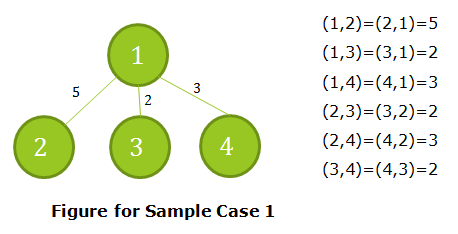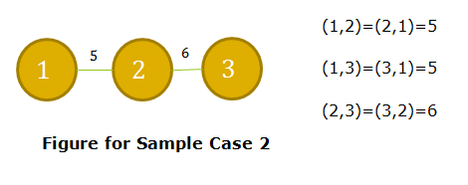# Flow on Tree

Limits 1s, 512 MB

Mr. Kaboom has recently learned about maximum flow. Now his friend Mr. Taboom gave him this problem.

You are given a tree with $N$ vertices. The vertices are numbered from 1 to $N$. Each edge of the tree has some capacity associated with it. Between each pair of vertices there is only one way to go.

Now imagine for each pair of vertices that one of the vertex is the source and the other is the sink. Then Mr. Kaboom needs to find out what is the maximum flow for each pair of vertices. Mr. Taboom decides to reduce Mr. Kaboom's misery and asked him to provide the sum of maximum flow between all possible pair of vertices.

Mr. Kaboom has found no solution and has trusted you with this task. Can you save his day?

## Input

The very first line of the input will contain the number of test cases $T$ ($1 ≤ T ≤ 20$). Then $T$ tests follow. First line of each test contains $N$ ($1 ≤ N ≤ 10^5$), the number of vertices in the tree. The next $N-1$ lines each contain three integers. The first two integers denotes the vertices this edge connects. The third integer gives the capacity of the edge.

The capacities of each edge will be non-negative and no more than $10^6$. And, the total number of vertices among all test cases will be less than $5×10^5$.

## Output

For each tests output one integer per line, the sum of all pair maximum flow in the tree.

See the explanation of the sample for more understanding. In every pair the vertices should be different.

## Sample

InputOutput
2
4
1 2 5
1 3 2
1 4 3
3
1 2 5
2 3 6

34
32


For the first case, the maximum flow for each pair is:For the second case, the maximum flow for each pair is:uDebug

### Submit